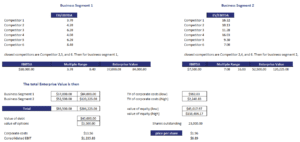# Sum Of The Parts Valuation (SOTP)

A sum of the parts valuation or break-up analysis is a valuation method that is used to analyse holding companies. Rather than trying to value the overall company, a sum of the parts valuation will determine the value of the different constituent companies or divisions. To value the divisions, analysts will estimate the value of the divisions if they would have to be spun off or sold to third parties.

On this page, we discuss the sum of the parts valuation approach and discuss a sum of the parts valuation example. The sum of the parts valuation implementation is done using an Excel spreadsheet. The spreadsheet is available for download at the bottom of this page.

## Sum of the parts definition

As the name suggests, a SOTP valuation breaks up a conglomerate into the distinct business units and tries to value the different units separately. Then the enterprise values of the different business units are aggregated to arrive at an estimate of the conglomerate’s value.

To perform a SOTP valuation, we should follow a number of steps:

1. Identify the divisions of the conglomerate or holding company.
2. Find the competitors for each of the divisions. To find the competitors, analysts will typically consult 10-K forms or Bloomberg
3. Determine the relevant valuation multiples. This depends on the sector that is being analyzed. For example, if the holding has a real estate division, different multiples are typically used for this division compared to a retail company. Very often enterprise value (EV) will be calculated for all divisions (see further).
4. Calculate the multiples (e.g. P/E, EBIT/sales, EV/sales,…) for both the competitors and the divisions. Use the results of the competitors to construct an interval.
5. Next, consider the centralized costs of the conglomerate. These are borne by all divisions and should be taken into consideration as well! To calculate centralised expenses, we do the following. Take the total EV, which is the sum of the EVs for the different divisions, and divide it by consolidated Earnings before interest and taxes (EBIT). Multiply this number by centralised cost c.

## Sum of the parts valuation Excel

Finally, we discuss an example of the SOTP valuation model using a simple spreadsheet. While the approach is fairly straightforward, collecting all the data can be a tedious job. Still, the example spreadsheet at the bottom of the page illustrates that one can easily do this type of valuation using some basic formula.Summary

we discussed the SOTP valuation model. It is commonly used to value conglomerates, holdings, and companies active in multiple industries.

### Sum of the Parts Valuation calculator

Want to have an implementation in Excel? Download the Excel file: Sum of the parts valuation